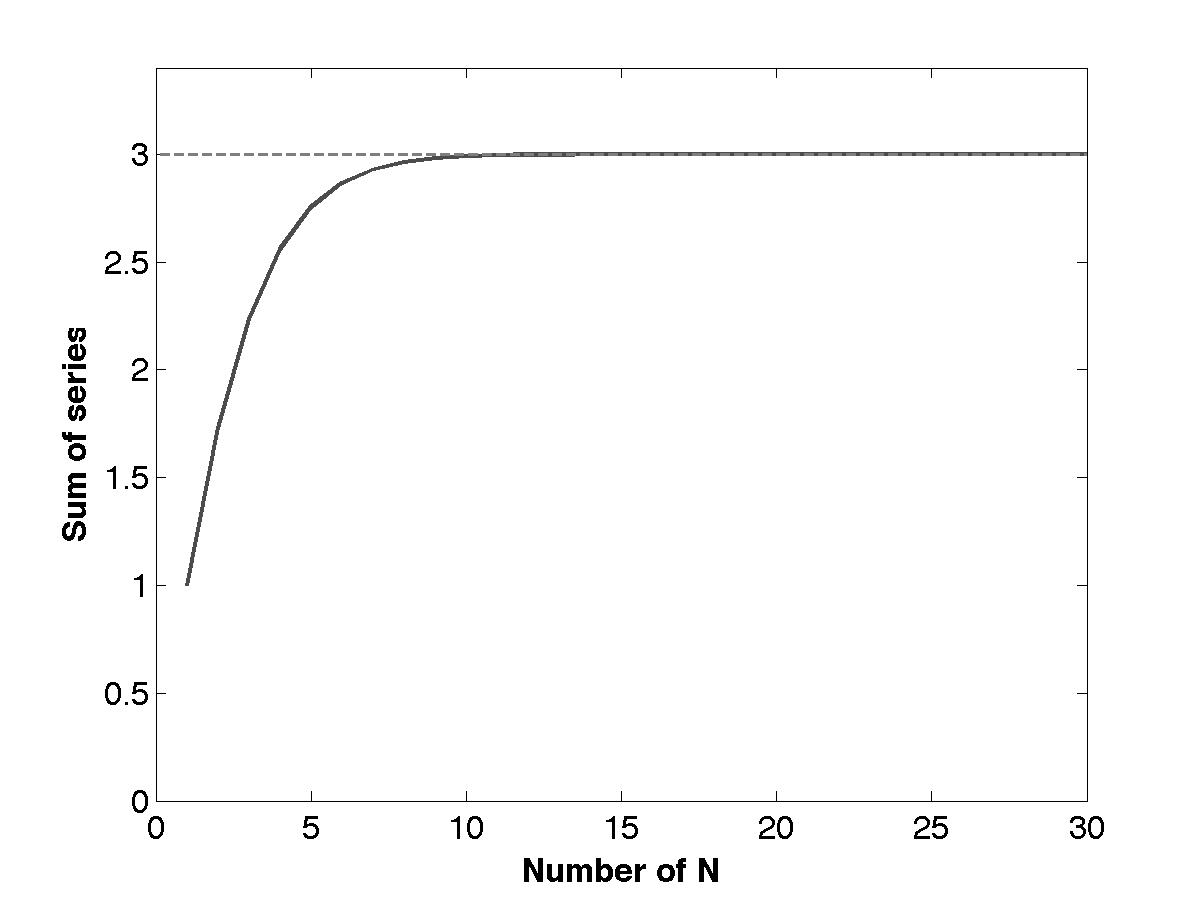In the biography of S. Ramanujan “The Man Who Knew Infinity: A Life of the Genius Ramanujan” (excellent, by the way), the author Robert Kanigel describes how Ramanujan’s first published work was a little problem he posed for other mathematicians. In it, Ramanujan asks for the value this series takes:

$\sqrt{1 + 2\sqrt{1 + 3\sqrt{1 + \dots}}}$

Nobody found a solution for six months, so he supplied a more general solution himself (see here and here) and the answer is 3.

Ramanujan filled his notebooks with numerical computations and some of his statements he did not prove, but were intuitions gained from his computational experiments. So, if we didn’t know the solution, could we simulate this series to get an idea of what it’s up to?

I think this naturally lend itself to recursion, so a function that calls itself. In Matlab (repo):

And call it with:

This converges fast:For every square root, we call iter_sqrt once. So the runtime is linear in $$N$$, $$\mathcal{O}(N)$$.

After writing this I saw that John D. Cook had written a similar post. And I found a flaw in my thinking. Because the cost for computing every element of the series is linear, but computing every consecutive element is more costly. The first element takes one calculation step, the second two and so on, so $$1 + 2 + \dots N = \frac{N(N+1)}{2}$$. Which means the full computation takes $$\mathcal{O}(N^2)$$. Cook writes:

I don’t see how to write a different algorithm that would let you compute f(n+1) taking advantage of having computed f(n). Do you? Can you think of a way to evaluate f(1), f(2), f(3), … f(N) in less than O(N$$^2$$) time?

I can think of two approaches to make this faster, but both fail. First, we cannot use f(1) to calculate f(2), f(3), …, because the true value of f(1) depends on what comes after. Instead f(1) is a crude approximation to f($$\infty$$).

Second, I thought, maybe we can flip this around. If we cannot find an algorithm which uses f(n) to calculate f(n+1), then maybe we can find an algorithm that just finds f(n) brute force and then infers what the previous values were. It is indeed possible to get the value of all the nested radicals from calculating f(n). But calculating f(1), f(2), …, f(n-1) from this still takes the same number of steps as before, so nothing is gained.